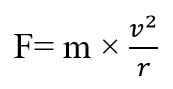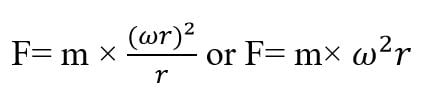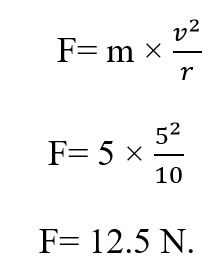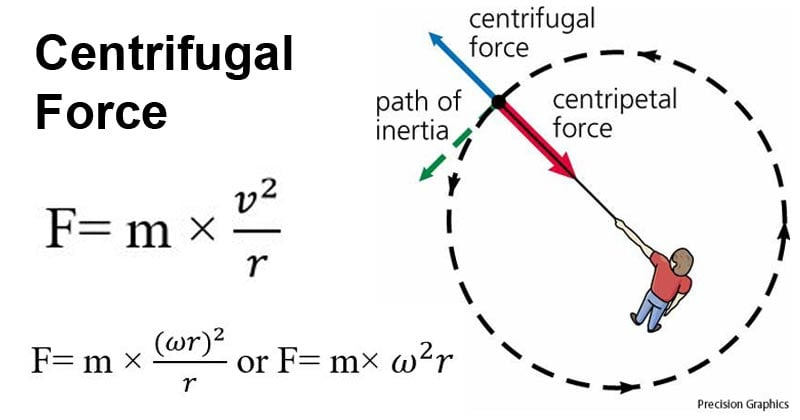# Centrifugal force- definition, formula, Principle, Examples (vs Centripetal force)

Last Updated on October 16, 2020 by Sagar Aryal

## Centrifugal force definition

Centrifugal force is an outward fictitious force that is experienced by an object moving in a circular path directed away from the center of rotation.

• The direction of this force is away from the axis of rotation and is parallel to the axis of rotation.
• Centrifugal force is equal in magnitude and dimensions with another force (centripetal) that acts towards the center of a circular path.
• It is termed a fictitious force because it only comes to play when there is a centripetal force. This force results due to the inertial property of the body moving in a circular path.
• The force does, however, depend on the mass of the object, the distance of the object from the center, and the speed of the rotation.
• The concept of centrifugal force has been used in various rotating devices like centrifuge rotors, banked roads, and centrifugal pumps.
• The unit of centrifugal force is Newton, and the dimensional formula is M1L1T-2.

## Centrifugal force formula

The formula to calculate the centrifugal force is given below.

If the velocity of the moving object is known, the centrifugal force can be calculated by the formula:where v is the velocity of the moving body, r is the distance of the moving body from the center and m is the mass of the moving body.

If the angular velocity of the moving object is known, the centrifugal force can be calculated by the formula:where ω is the angular velocity, r is the distance of the moving body from the center and m is the mass of the moving body.

## Principle of Centrifugal force

• As discussed above, the centrifugal force is fictitious or pseudo as it only exists when a centripetal force is present.
• When a car with passengers turns the wheel, the vehicle is driven by the centripetal force that acts on all parts of the vehicle.
• However, the passengers in the car retain the freedom of their movement and thus maintain a straight path when the vehicle begins to turn.
• This causes the passengers to move towards the edge of the seat towards the door, which transmits the centripetal force to the passengers.
• The force experienced by the passengers away from the car is the centrifugal force.
• Newton’s law of motion doesn’t consider this a real force as according to Newton’s second law of motion, acceleration is caused by force.
• When a stone at the end of the string tied to a pole on the ground is rotated, the direction of the velocity of the stone changes continuously, which creates acceleration.
• If the string breaks, the stone tends to retain its inertia and move in a straight line tangent to the circular path.
• If the centrifugal force was real, the stone would move in an outwards direction instead of moving tangentially.

## How is centrifugal force calculated?

For this, let us take the same example of stone at the end of the string tied to a pole. In order to calculate the centrifugal force experienced by the stone, the following steps can be followed:

1. Determine the mass (m) of the stone. Let’s say the mass is 5 kg.
2. Determine the length of the string. Let’s say the length is 10 m.
3. Determine the velocity of the stone. Let’s assume that the tangential force is 5 m/s. The velocity can be either tangential velocity (v) or angular velocity (ω). If the velocity is the angular velocity, the tangential velocity can be calculated as :

### v = ω × r

1. Calculate the centrifugal force by the given formula:Thus, the centrifugal force will be 12.5 N.

## Applications of Centrifugal force

Even though Newton’s law doesn’t consider the centrifugal force a real one, it has multiple applications:

1. Centrifuges operate on the principle of centrifugal force. The centrifugal force created due to the rotors induces a hydrostatic pressure gradient in the tubes directed perpendicular to the axis of rotation. This results in larger buoyant forces that push the less dense particles inwards while the denser particles are moved outwards. This principle allows the separation of particles on the basis of their densities.
2. The centrifugal governor is a system that maintains a constant speed in an engine by moving the fuel or working liquid radially which is caused by the centrifugal force.
3. Centrifugal forces are used to generate artificial gravity in rotating space stations. These stations help to study the effects of gravity of other plants in a simulated way.
4. Centrifugal force is also used by washing machine dryers where the spinning of the rotor in a washing machine generates a centrifugal force that causes the clothes to move away from the center. This causes the water to be forced out of the wet clothes through the holes present in the chamber.
5. Centrifugal forces are used in various rides in amusement parks where the force pushes the riders against the wall and allows the passengers to be raised above the floor of the machines.

## Examples of Centrifugal force

Centrifugal force can be seen in various process in our daily life; some examples are given below:

1. The force acting on the passengers outwards in a car when the car is taking a turn is an example of centrifugal force.
2. When a stone tied to a string is whirled in a circle, the force exerted on the hands is also because of centrifugal force.
3. The earth is flattened at the pole and bulged at the equator because the centrifugal force acting on the particles in the equator is maximum.
4. When a bucket filled with water is rotated in a circle, the water in the bucket doesn’t fall because the weight of the bucket is balanced by the centrifugal force acting on the bucket.
5. The mud stuck on the wheels of cars is thrown tangentially towards the mudguard as a result of centrifugal force.Image Source: Wikia.

## Centrifugal force vs Centripetal force

 Basis of comparison Centripetal force Centrifugal force Definition Centripetal force is the force acting on a body moving in a circular path along the radius of the circular path and is directed towards the center of the circle. Centrifugal force is an outward fictitious force that is experienced by an object moving in a circular path directed away from the center of rotation. Nature It is a real force and has real effects. It is considered a fictitious or pseudo force but has real effects. Direction It is directed towards the center of the circle of rotation. It is directed away from the center of the circle of rotation. Role A circular motion doesn’t exist without centripetal force. Centrifugal force doesn’t have an independent existence. Origin The origin of the centrifugal force is due to the interaction between two objects. The origin of the centrifugal force is due to inertia. FormulaAction The centripetal force acts in both inertial and non-inertial frames. Centrifugal force acts only in the rotating frame (non-inertial frames).

## References and Sources

• Khatry MK et al. Principles of Physics. Aayam Publications.
• 3% – https://thefactfactor.com/facts/pure_science/physics/centripetal-force/6311/
• 2% – https://en.wikipedia.org/wiki/Centrifugal_force_(rotating_reference_frame)
• 1% – https://www.scienceabc.com/nature/what-is-centripetal-acceleration-what-is-centrifugal-force.html
• 1% – https://www.sarthaks.com/204136/stone-tied-string-length-whirled-vertical-circle-with-the-other-end-the-string-the-centre
• 1% – https://www.reference.com/science/centripetal-force-involved-operation-washing-machine-ee15394203b35628
• 1% – https://www.britannica.com/science/centrifugal-force
• 1% – https://wikimili.com/en/Centrifugal_force
• 1% – https://thenewtonslaw.com/what-is-newtons-second-law-of-motion/